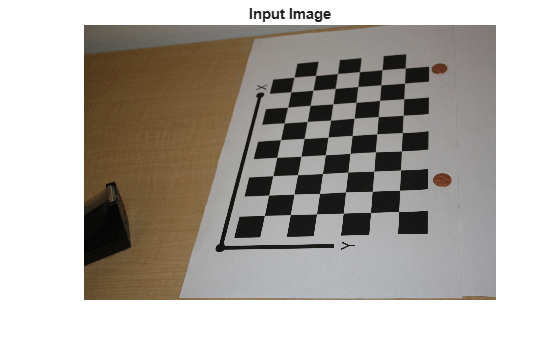# cameraProjection

Camera projection matrix

Since R2022b

## Syntax

``camProjection = cameraProjection(intrinsics,tform)``

## Description

example

````camProjection = cameraProjection(intrinsics,tform)` returns a 3-by-4 camera projection matrix `camProjection`. You can use `camProjection` to project a 3-D world point in homogeneous coordinates into an image according to the transformation `tform`.```

## Examples

collapse all

Create a set of calibration images.

```images = imageDatastore(fullfile(toolboxdir("vision"),"visiondata", ... "calibration","slr"));```

Detect the checkerboard corners in the images.

`[imagePoints,boardSize] = detectCheckerboardPoints(images.Files);`

Generate the world coordinates of the checkerboard corners in the pattern-centric coordinate system, with the upper-left corner at (0,0). The square size is in millimeters.

```squareSize = 29; worldPoints = generateCheckerboardPoints(boardSize,squareSize);```

Calibrate the camera.

```I = readimage(images,1); imageSize = [size(I,1) size(I,2)]; cameraParams = estimateCameraParameters(imagePoints,worldPoints,ImageSize=imageSize); intrinsics = cameraParams.Intrinsics;```

Load an image at a new location.

```imOrig = readimage(images,9); figure imshow(imOrig) title("Input Image")```Undistort the image.

`[im,newOrigin] = undistortImage(imOrig,intrinsics,OutputView="full");`

Find the reference object in the new image.

`[imagePoints,boardSize] = detectCheckerboardPoints(im);`

Compensate for the image coordinate system shift.

`imagePoints = imagePoints+newOrigin;`

Calculate new extrinsics.

`camExtrinsics = estimateExtrinsics(imagePoints,worldPoints,intrinsics);`

Calculate the camera projection matrix.

`P = cameraProjection(intrinsics,camExtrinsics)`
```P = 3×4 105 × 0.0157 0.0404 0.0199 8.9399 -0.0271 -0.0046 0.0387 9.4399 0.0000 -0.0000 0.0000 0.0072 ```

## Input Arguments

collapse all

Camera intrinsics, specified as `cameraIntrinsics` object. The object stores information about a camera’s intrinsic calibration parameters, including the lens distortion parameters.

Transformation from world coordinates to camera coordinates, specified as a `rigidtform3d` object. You can use the `estimateExtrinsics` function to create the `tform` object. The `R` and `Translation` properties of the object represent the rotation matrix and translation needed to project 3-D world points in homogeneous coordinates into an image.

## Output Arguments

collapse all

Camera projection matrix, returned as a 3-by-4 matrix. The matrix contains the 3-D world points in homogenous coordinates that are projected into the image.

The function calculates `camProjection` using the intrinsic matrix K and the `R` and `Translation` properties of the `tform` object as follows:

`camProjection` = K × `[tform.R tform.Translation']`

Using the camera projection matrix and homogeneous coordinates, you can project a world point onto the image.

w × [x,y,1]' = `camProjection`× [X,Y,Z,1]'

 (X,Y,Z) are the world coordinates of a point (x,y) are the coordinates of the corresponding image point w is an arbitrary scale factor

When the `R` and `Translation` properties of the transformation `tform` are of data type `double`, the function returns `camProjection` as `double`. Otherwise the function returns `camProjection` as `single`.

Data Types: `single` | `double`

## Version History

Introduced in R2022b

expand all